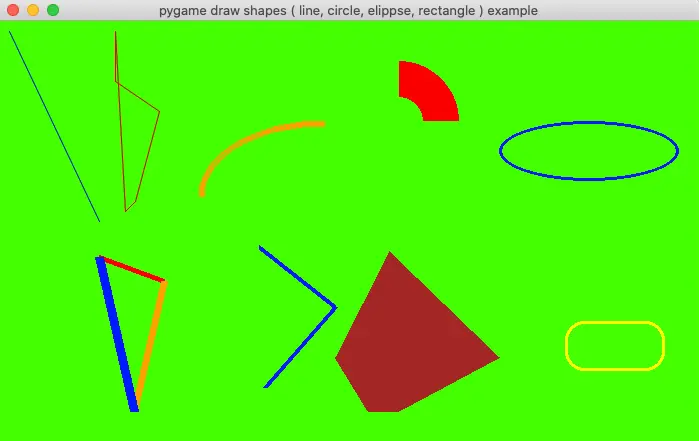# How To Draw Shapes ( Line, Circle, Arc, Ellipse, Rectangle ) In Pygame

This article will tell you how to draw multiple shapes in pygame application with examples. It will use the multiple functions in the pygame.draw module, we will introduce them one by one.

### 1. How To Use The Functions In pygame.draw Module To Draw Different Shapes.

1. pygame.draw.aaline(surface, color, start_pos, end_pos, blend=1) -> Rect: This function draw only one straight antialiased line.
2. pygame.draw.aalines(surface, color, closed, points) -> Rect: This function can be used to draw mulitple antialiased lines.
3. pygame.draw.arc(surface, color, rect, start_angle, stop_angle, width=1) -> Rect: This function can draw an arc line.
4. pygame.draw.circle(surface, color, center, radius, width=0, draw_top_right=None, draw_top_left=None, draw_bottom_left=None, draw_bottom_right=None) -> Rect: Draw a circle with provided parameters.
5. pygame.draw.ellipse(surface, color, rect, width=0) -> Rect: Draw an ellipse shape.
6. pygame.draw.line(surface, color, start_pos, end_pos, width=1) -> Rect: Draw a line.
7. pygame.draw.lines(surface, color, closed, points, width=1) -> Rect: Draw multiple lines.
8. pygame.draw.polygon(surface, color, points, width=0) -> Rect: Draw a polygon with provided parameters.

### 2. How To Draw Multiple Shapes In Pygame Application Examples.

1. Below are the examples of the above function, you can read the code comments for a detailed explanation.
2. The python source file name is PygameDrawShapes.py, below is the file content.
```'''
Created on Feb 7, 2022

@author: songzhao
'''
import sys, math, pygame

# declare a global variable to save the pygame main window surface object.
main_window_surface = None

# initialize the pygame application.
def initialize_pygame():

pygame.init()

global main_window_surface

# create the pygame application's main window surface object.
main_window_surface = pygame.display.set_mode(size = (700, 500), flags = pygame.RESIZABLE)

# set the window title.
pygame.display.set_caption('pygame draw shapes ( line, circle, elippse, rectangle ) example')

# fill the window background color.
main_window_surface.fill(pygame.Color('green'))

# draw one antialias line.
def draw_aaline():

pygame.draw.aaline(surface = main_window_surface, color = pygame.Color('blue'), start_pos = (10, 10), end_pos = (100, 200))

# draw multiple antialias lines.
def draw_aalines():

pygame.draw.aalines(surface = main_window_surface, color = pygame.Color('red'), closed = True, points = ((116, 10), (116, 60), (160, 90), (136, 180), (126, 190)))

# draw an arc line.
def draw_arc():

pygame.draw.arc(main_window_surface, pygame.Color('orange'), (200,100,250,150), math.pi/2, math.pi, width=6)

# draw a partial red circle.
def draw_circle():

pygame.draw.circle(surface = main_window_surface, color = pygame.Color('red'), center = (400, 100), radius = 60, width = 36, draw_top_right = True)

# draw an ellipse shape.
def draw_ellipse():

pygame.draw.ellipse(main_window_surface, pygame.Color('blue'), (500, 100, 180, 60), 3)

# draw three separate single line.
def draw_line():

pygame.draw.line(main_window_surface, pygame.Color('red'), (100, 236), (165, 260), 5)

pygame.draw.line(main_window_surface, pygame.Color('orange'), (165, 260), (135, 390), 7)

pygame.draw.line(main_window_surface, pygame.Color('blue'), (135, 390), (100, 236), 9)

# draw multiple lines use one function.
def draw_lines():

'''
If you do not provide the points keyword argument, it will throw the below error message.

TypeError: points argument must be a sequence of number pairs.
'''
pygame.draw.lines(main_window_surface, pygame.Color('blue'), closed = False, points = ((260, 226), (336, 286), (266, 366)), width = 5)

# draw a brown color polygon.
def draw_polygon():

pygame.draw.polygon(main_window_surface, pygame.Color('brown'), ((390, 230), (500, 336), (399, 390), (369, 390), (336, 336)))

# draw a yellow rectangle with provided parameters width, border radius etc.
def draw_rectangle():

pygame.draw.rect(surface = main_window_surface, color = pygame.Color('yellow'), rect = (566, 300, 100, 50), width = 3, border_radius = 19)

# this function listen and handle the pygame application's events.
def main_loop():

while True:

for event in pygame.event.get():

if event.type == pygame.QUIT:

exit()

# in this function, if the surface_object is not None,
# it will draw the target_surface_object to the source surface_object at the specified location.
def update_screen(surface_object, target_surface_object, top_left_corner_x, top_left_corner_y):

if surface_object != None:

surface_object.blit(target_surface_object,(top_left_corner_x, top_left_corner_y))

pygame.display.update()

if __name__ == '__main__':

# initialize the pygame application.
initialize_pygame()

# draw multiple shapes.
draw_aaline()

draw_aalines()

draw_arc()

draw_circle()

draw_ellipse()

draw_line()

draw_lines()

draw_polygon()

draw_rectangle()

# update the screen to show the above shapes.
update_screen(None, None, None, None)

main_loop()
```
3. When you run the above example source code, you can get the below picture.This site uses Akismet to reduce spam. Learn how your comment data is processed.